20 Great Ways To Learn Multiplication Facts - Times Tables

Welcome to my second blog in my new series focusing on how to support the older child in Primary school. In this blog I share 20 great ways that can help children learn multiplication facts, or more commonly known as times tables.

Let me point out from the beginning, that before helping your child to remember multiplication facts, it is essential that they have an understanding of the concept of multiplication. Multiplication and division can be taught together as they are the opposite operations of each other. Children need to experience both operations together to gain a deep knowledge about the idea of multiplying and dividing.

It is important to expose your child to a variety of terms relating to multiplication so they have a greater vocabulary when it comes to mathematics. Below are examples of two concepts that can be used in multiplication problems.

“Groups of”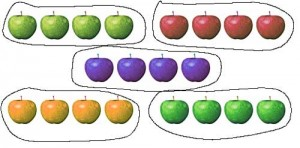This is when objects are placed in groups. You can multiply the amount of objects in one group by the amount of groups.

“Rows of”This is when objects are placed in rows. You can multiply the amount of objects in one row by the amount of rows.

Children need to understand the both concepts above as well as knowing the terms multiply, times, product (answer to a multiplication problem) and factors (the numbers that are multiplied together).

Here are some strategies that your child may use to work out a multiplication problem (3 x 5=) before the facts become known. These are the strategies taught in younger years.

1. Repeated addition : 5 + 5 + 5 = 15   or   3 + 3 + 3 + 3 + 3 = 15

2. Skip count: 5, 10, 15      or       3, 6, 9, 12, 15

3. Use a times table chart

5. Draw an array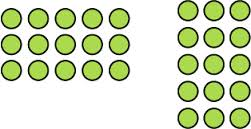Once the child has a clear understanding of multiplication, it is then time to learn all their facts. Most teachers set a particular times table per week that the children must revise. Below is a list of 20 ways to help children remember multiplication facts.

1. Memory GameWrite a multiplication question on one card and the answer on another. Do this for as many facts as you want. Turn all the cards over and your child needs to find the matching cards.

2. Nine times table trick up to times by ten.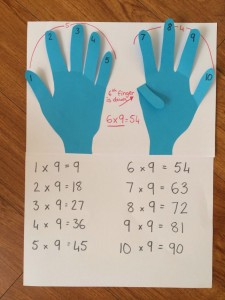Have your child put all their fingers up. If the question is 9 x 6, they put their sixth finger down. The answer is shown on their fingers. The amount of fingers in front of the finger that is down is the tens number and the amount of fingers after the finger that is down is the ones number. Therefore the answer is 54.

3. Dominoes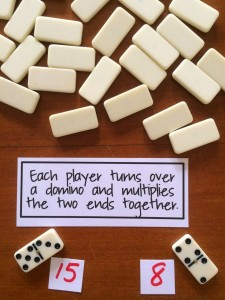Turn all the dominoes over. Take turns flipping the dominoes over and multiplying the two numbers. You can make it harder by flipping one domino over and adding the dots together and turning over another domino and adding those dots together. Then you could multiply the two higher numbers together.

4. Multiplication jumping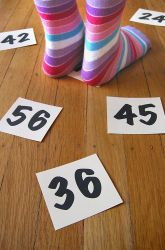Have all the answers of a particular times table set (eg 5 times tables) written on different cards (or the facts that your child struggles with remembering). Spread them out on the floor. Call out a multiplication question, eg 5 x 6 and the child has to quickly find the answer and jump on it.

5. Dice roll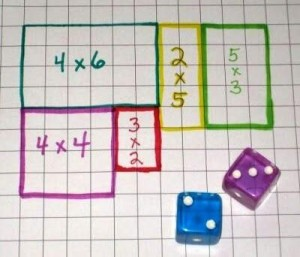Print off some grid paper or use grid paper from a maths book. Roll two dice and draw the rectangle or square on the grid paper. Your child then writes the multiplication fact and answer inside the shape.

6. Egg Carton Multiplication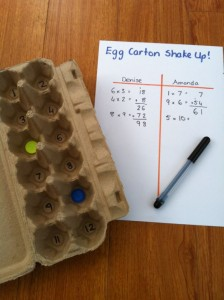Write the numbers one to twelve in each hole of an empty egg carton. Put two objects inside the carton and close the lid. Shake the egg carton up and open it. The child says the multiplication question and answer depending on what hole the objects are in. Eg 4 x 3 = 12

7. Karate belts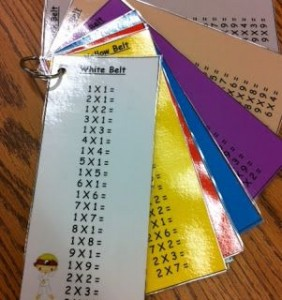Get your child to pretend they are a multiplication ninja. In order to get their new belt they must be able to answer all their multiplication facts set on that particular belt. All the multiplication facts for a set (eg 7 times tables) must be in random order to avoid the child just skip counting.

8. Groups of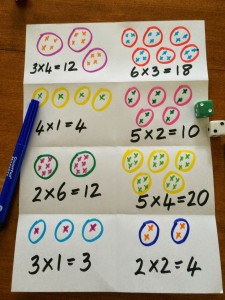Roll the dice and record the multiplication fact using a “groups of” representation. They could do the same for a “rows of” representation (also known as an array).

9. Multiplies game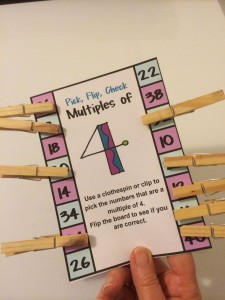Make a card with a number in the middle. On the outside of the card put lots of different numbers (some multiplies of the number and some not). Your child then uses pegs to pick all the correct multiplies. You can list the correct numbers on the back of the card.

10. Multiplication JengaWrite multiplication questions and stick on the side of slim building blocks. Stack the blocks up (3 going one way and then the next three blocks going the other way - just like Jenga) Your child takes time to slide out one of the blocks without knocking down the tower. They need to answer the multiplication fact on the block they got out.

11. Multiplication board game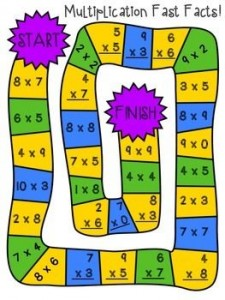Make up a board game with multiplication questions on each square. If your child lands on a square they need to answer the question before they roll for their next go.

12. Multiply cut outs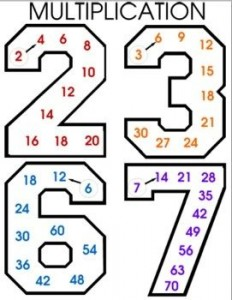Use large number cutouts and a texta. Your child writes all the multiplies or multiplication facts associated with that number on the inside.

13. Number fishingUse magnetic numbers and a string with a paper clip on it. Your child puts the “fishing line” into a bucket with the magnetic numbers and “catches” a number. They need to repeat this to get a second number. They can then multiply the two numbers together.

14. Multiplication Bingo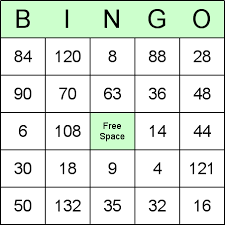Each child has a bingo card with numbers that will become the answers to multiplication facts. Play the game with one child or a few children. If playing with a few, make sure they all have different bingo cards. The caller reads out a multiplication fact and if the answer in on the card, the player puts an object on it. Once all numbers on the card have objects on it, the child can call out “Bingo”.Play snakes and ladders with a twist. Each square will have a multiplication fact that the child must answer before they have their next go.

16. Multiplication soccerWrite numbers all over a soccer ball. The child throws the ball up and catches it. They look at which numbers their thumbs are touching and multiply them together. If they get the correct answer they can shoot the ball 2 metres from the goal post. If they get it in the goal, they move back another 2 metres and answer a new multiplication fact. The aim is to get back as far as they can. If they get the answer wrong or miss the goal, they must start again. For every correct answer they move 2 metres further away from the goal.

17. Multiplication Battle Ships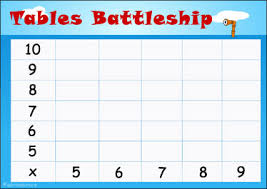Use a battle ship card that has an x and y axis with numbers on each axis. Get each player to draw some battle ships that are 1 ship that is 3 squares long, 2 ships that are 2 squares long and 3 ships that are 1 square long (altogether there would be 6 ships). Each player takes turns to try and work out where the other players ships are without looking. They ask if there is a ship at 6  x 8  position as well as saying the answer. Always start with the y axis. The player puts a counter on successful hits. Whoever sinks all the ships first is the winner.

18. Pack of cards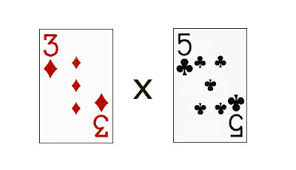Shuffle a deck of cards and split in half. Turn over a card from each pile and multiply them together. Jacks represent 10, Queens represent 11, Kings represent 12 and Aces represent 1.

19. Multiplication SongsThere are a variety of multiplication songs out there. Get your child to listen to a song for each set of times tables. You can listen to these songs through youtube or buy a CD online.

20. Multiplication with Clocks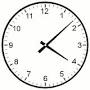Get your child to look at the clock and state the numbers that the hands are pointing to. Multiply them together and say the answer. It would be great to use a clock where a partner can turn the hands to various numbers.

Hopefully these 20 ideas will help your children to be engaged and enthusiastic towards learning their multiplication facts. Knowing multiplication facts will help children solve mathematical problems. Ensure that your children have a solid understanding of multiplication before rote learning the facts.

Please share this blog if you have found it insightful and if you believe it offers practical information that your can implement at home or in the classroom. Remember to email a copy of it to yourself for reference later on.

Kelly Pisani